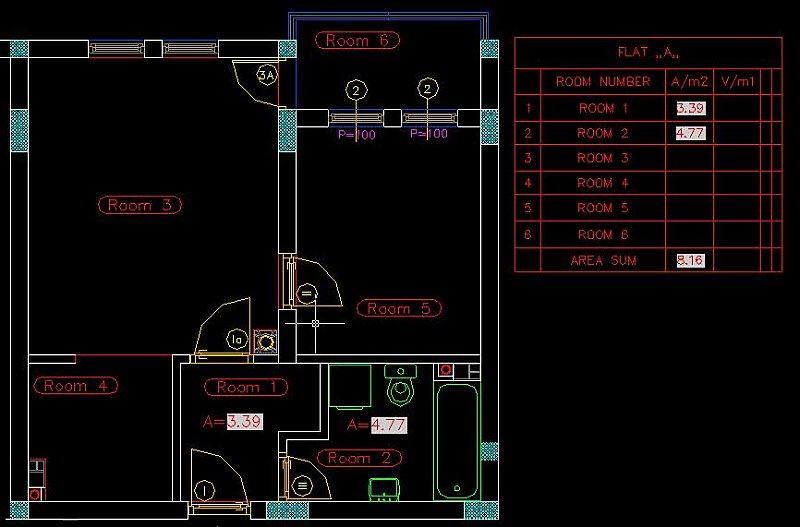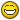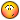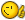# Fill table cells with fields

## Recommended PostsI need quickiest way to fill table cells with fields.

For instance, if I have 5 rooms I need for each room to find area with field and to copy field content in a table cell.

I use lisp functions that create MTExt with area field and put it in a room with area of that room. If I would like to copy field into cell I need to get in MText with double click, copy field content and then select cell and copy content in it.

I would like with lsp not to create MText entity containing the field but to put field expresion into cell, then to select another room etc.

To be clear, I need in loop to find field area, select cell and put field in cell.

```(defun c:AREACELL ()
(setq *model-space*
(vla-get-ModelSpace
)
)
;;pass this function an entity and a point
(defun LinkedArea (ent pt / obj objID ip width str)
;;convert the entity to an object
(setq obj (vlax-ename->vla-object ent)
objID (vla-get-objectid obj)
ip (vlax-3D-Point pt)
width 0.0
;;set the string - this creates the field
str (strcat
"%<\\AcObjProp.16.2 Object(%<\\_ObjId "(rtos objID 2 2)">%).Area \\f
\"%lu2%pr2%ct8[0.0001]\">%"
)
)
;;Create the MTEXT entity containing the field.
)
(while
;; Set a = the entity and set b = Point for text
(setq ent (entsel "\n Pick Object or Enter for exit"))
(setq a (car ent))
(setq b (getpoint "\n Point for text: "))

;;Call the function
)
(princ)
)
```

##### Share on other sitesIf it is necessaryto explain more, just ask.

Help me, please, I need it today!

##### Share on other sitesa Drawing of this will helps a lot

##### Share on other sitesOK, I'll post a drawing for a hour at least, but I desperitely need help.

I think this is not too complicate as it seems to be.

##### Share on other sitesHere is a picture.

I'll try to explain what I want.

I have a drawing with flats, so I need for each flat to fill table with area of each room using field because, often, I need to change some room so area will change too.

So, each room has polyline that represent that room. I want to start "lisp tool" to select one room, grab area field, select cell in a table for flat "A" and put field in a cell, then select another room etc.

Now, with lisp program AreaCell.lsp I select room and put mtext with a field (A=3.39 for room1), then double click on mtext, select just a field, copy field in clipboard, select cell in table for flat "A" and paste from clipboard.

I think, now is very clear what I want.##### Share on other sites##### Share on other sitesThis will put your fields into cells in a table.

• Select your rooms in turn.
• Click inside the cell you want the field in.

```(defun c:sCell (/ tab ent Obj pt tObj row col)
(if (and (setq tab (car (entsel "\nSelect Table: ")))
(eq "ACAD_TABLE" (cdr (assoc 0 (entget tab)))))
(while
(and
(setq ent (car (entsel "\nSelect Room: ")))
(vlax-property-available-p
(setq Obj
(vlax-ename->vla-object ent)) 'Area))
(while
(progn
(setq pt (getpoint "\nClick into Cell to place field: "))
(cond ((vl-consp pt)
(if (eq :vlax-true
(vla-hittest
(setq tObj
(vlax-ename->vla-object tab))
(vlax-3D-point pt)
(vlax-3D-point (trans '(0 0 1) 0 1)) 'row 'col)) nil
(princ "\n** No Cell Selected **")))
(t (princ "\n** No Point Selected **")))))
(vla-setText tObj row col
(strcat
"%<\\AcObjProp Object(%<\\_ObjId "
(vl-princ-to-string
(vla-get-Objectid Obj))
">%).Area \\f \"%lu2%pr2\">%")))
(princ "\n** No Table Selected **"))
(princ))

```

##### Share on other sitesLeeMac, what to say. I am aspolutely satisfied with your solution. When I cry for help I hope that you will help me as your are.

Thank you very much, you are so helping me these days.##### Share on other sitesLeeMac, what to say. I am aspolutely satisfied with your solution. When I cry for help I hope that you will help me as your are.

Thank you very much, you are so helping me these days.Happy to help - glad it was what you wanted##### Share on other sitesHappy to help - glad it was what you wantedLeeMacc, maybe your happines will not be so high when I ask you for small modification.

I need to put Area and Length of Room in two neighbour columns in a same table so it would be perfect, if it is possible, to do same as you describe but with resulting in one more field at the right of the Area field (with one click in a cell to put Area and Length field).

Sorry to bother you.##### Share on other sitesLeeMacc, maybe your happines will not be so high when I ask you for small modification.

I need to put Area and Length of Room in two neighbour columns in a same table so it would be perfect, if it is possible, to do same as you describe but with resulting in one more field at the right of the Area field (with one click in a cell to put Area and Length field).

Sorry to bother you.Ok, will see what I can do##### Share on other sitesTry this:

```(defun c:sCell (/ tab ent Obj pt tObj row col)
(if (and (setq tab (car (entsel "\nSelect Table: ")))
(eq "ACAD_TABLE" (cdr (assoc 0 (entget tab)))))
(while
(and
(setq ent (car (entsel "\nSelect Room: ")))
(vlax-property-available-p
(setq Obj
(vlax-ename->vla-object ent)) 'Area)
(vlax-property-available-p Obj 'Length))
(while
(progn
(setq pt (getpoint "\nClick into Cell to place field: "))
(cond ((vl-consp pt)
(if (eq :vlax-true
(vla-hittest
(setq tObj
(vlax-ename->vla-object tab))
(vlax-3D-point pt)
(vlax-3D-point (trans '(0 0 1) 0 1)) 'row 'col)) nil
(princ "\n** No Cell Selected **")))
(t (princ "\n** No Point Selected **")))))
(vl-catch-all-apply
(function
(lambda ( )
(vla-setText tObj row col
(strcat
"%<\\AcObjProp Object(%<\\_ObjId "
(vl-princ-to-string
(vla-get-Objectid Obj))
">%).Area \\f \"%lu2%pr2\">%"))
(vla-setText tObj row (1+ col)
(strcat
"%<\\AcObjProp Object(%<\\_ObjId "
(vl-princ-to-string
(vla-get-Objectid Obj))
">%).Length \\f \"%lu2%pr2\">%"))))))
(princ "\n** No Table Selected **"))
(princ))
```

##### Share on other sitesThat's it LeeMac.Well done.

But shame on me.

Sometimes, when you just try to do something before asking for help, will probably successed in finding solution. But, I didn't try.

Thanks again Lee Mac

##### Share on other sitesThats Ok, it wasn't too hard to add the Length part, I enclosed it in a vl-catch-all-apply in case the table didn't have enough columns, or you clicked the last column.Lee

##### Share on other sitesMy routine.

This command allows to insert into the specified point of drawing or the specified cell of the table the text with a field (FIELD), containing value of the area of the constructed or chosen contour.

> Lee Mac

In my variant it is not necessary to choose the table. The text "hangs" on the cursor and hit in a table cell is analyzed. The core has allocated red.

```; A command: PAREATLB
; This command allows to insert into the specified point of drawing or the specified cell of the table
; The text with a field (FIELD), containing value of the area of the constructed or chosen contour.
; Accuracy of a rounding off and scale factor are adjusted through the Installation option
; As this field is connected with concrete object, at change
; Object the field is recalculated (field updating) is necessary
; The code can be kept in a file pareatlb.lsp
; Possible script for the button or menu point:
; ^C^C (if (not C:PAREATLB) (load "pareatlb")); PAREATLB;
;; the Variant scritp for the task м2
;; ^C^C (if (not C:PAREATLB) (load "pareatlb")); PAREATLB; S; 0.001; 2; 5;; м2;
;; Where
;; 0.001 - scale factor
;; 2 - accuracy of a rounding off
;; 5 - text height
;; the prefix is not present
;; м2 - a suffix

(defun C:PAREATLB ( / en cmdname fld txt fc tblset tblobj row col pt
;;;Return 2004 2005 2006 2007 2008
(setq Aver (atof (substr (getvar "ACADVER") 1 4)))
(cond ((= Aver 18.0) 2010)((= Aver 17.2) 2009)
((= Aver 17.1) 2008)((= Aver 17.0) 2007)((= Aver 16.2) 2006)
((= Aver 16.1) 2005)((= Aver 16.0) 2004)((= Aver 15.06) 2002)
(t 0)))
(or *SCALE* (setq *SCALE* 1))(or *PREC* (setq *PREC* 2))
(or *TEXTSIZE* (setq *TEXTSIZE* (getvar "TEXTSIZE")))
(or *SUFF* (setq *SUFF* ""))(or *PREF* (setq *PREF* ""))
(setq *SUFF* (vl-princ-to-string *SUFF*))
(setq *PREF* (vl-princ-to-string *PREF*))
(princ "\nCurrent scale = ")(princ *SCALE*)
(princ " Current accuracy of a rounding off = ")(princ *PREC*)
(princ " Text height = ")(princ *TEXTSIZE*)
(princ " Prefix= ")(princ *PREF*)(princ " Suffix= ")(princ *SUFF*)
(initget "Polyline Setting sElect")
(and
(or
)
(or ;_ >
(while (= (setq cmdname (getkword "\nSelect or Draw [Polyline/Setting/sElect] <select>: "))
"Setting")
(princ "\nNew scale <")(princ *SCALE*)(princ "> : ")
(initget 6)
(if (setq en (getdist))(setq *SCALE* en))
(princ "\nRounding off <")(princ *PREC*)(princ "> : ")
(initget 4)
(if (setq en (getint))(setq *PREC* en))
(princ "\nText height <")(princ *TEXTSIZE*)(princ "> : ")
(initget 6)
(if (setq en (getdist))(setq *TEXTSIZE* en))
(princ "\nPrefix (space - clear) <")(princ *PREF*)(princ "> : ")
(setq en (getstring t))(if (= en "")(setq en *PREF*))
(if (= en " ")(setq en ""))
(if (= (substr (vl-string-left-trim "\/" en) 1 2) "U+")
(setq en (strcat "\\" (vl-string-left-trim "\/" en))))(setq *PREF* en)
(princ "\nSuffix (space - clear) <")(princ *SUFF*)(princ "> : ")
(setq en (getstring t))(if (= en "")(setq en *SUFF*))
(if (= en " ")(setq en ""))
(if (= (substr (vl-string-left-trim "\/" en) 1 2) "U+")
(setq en (strcat "\\" (vl-string-left-trim "\/" en))))(setq *SUFF* en)
(initget "Polyline Setting sElect")
)
t
)
(cond
((= cmdname "Polyline")(setvar "CMDECHO" 1)(command "_.PLINE")
(while (> (getvar "CMDACTIVE") 0)(command pause))
(setq en (entlast))
)
((or (null cmdname)(= cmdname "sElect"))
(princ "\nSelect polyline, circle, spline, ellipse or arc")
(and
(setq tblset (ssget "_:S:E" '((0 . "*POLYLINE,ARC,SPLINE,ELLIPSE,CIRCLE"))))
(setq en (ssname tblset 0))
)
)
(t nil)
)
;_ Make Field
(setq fld (strcat "%<\\AcObjProp Object(%<\\_ObjId "
(vl-princ-to-string(vla-get-objectid (vlax-ename->vla-object en)))
">%).Area \\f \"%lu2%ps["*PREF* "," *SUFF*
"]%pr"(itoa *PREC*) "%ct8["(vl-princ-to-string *SCALE*)"]\">%"
) ;_ strcat
) ;_ setq
(setvar "cmdecho" 0)
(setq tstyle (getvar "TEXTSTYLE"))
;_ Создаем текст
(if (= (cdr (assoc 40 (tblsearch "STYLE" tstyle))) 0.0)
(vl-cmdf "_.-TEXT" "_S" tstyle "_none" '(0 0 0) *TEXTSIZE* 0 fld)
(vl-cmdf "_.-TEXT" "_S" tstyle "_none" '(0 0 0) 0 fld)
) ;_ end of if
(setq txt (entlast))

;_ We copy in the buffer and back
(vl-cmdf "_updatefield" txt "")
(princ "\n Specify a point of an insert of the text or a table cell:")
(vl-cmdf "_.copybase" (trans '(0 0 0) 0 1) txt "" "_.erase" txt "" "_.pasteclip" "_none" pause)
;_ In txt a text primitive thing in pt an insert point
(setq txt (entlast) pt (getvar "LASTPOINT"))
(or
[color="Red"] (and ;_We check, whether the point has got to a table cell
(setq  tblobj nil tblset (ssget "_X" '((0 . "ACAD_TABLE"))))
(setq lst (mapcar 'vlax-ename->vla-object(vl-remove-if 'listp (mapcar 'cadr (ssnamex tblset)))))
(mapcar '(lambda (x)
(or tblobj
(and
(= :vlax-true (vla-HitTest x
(vlax-3d-point (trans pt 1 0))
(vlax-3d-point (trans (getvar "VIEWDIR") 1 0))
'row 'col))
(setq tblobj x)
)
)
)
lst)
tblobj row col
(or (vla-SetText tblobj row col fld) t)
(entdel txt)
)[/color]
(and ;_Has not got, we draw the text with a field
(setq txt (vlax-ename->vla-object txt))
(vlax-write-enabled-p txt)
(vlax-method-applicable-p txt 'FieldCode) ;_FieldCode
(vlax-property-available-p txt 'TextString)
(vlax-put txt 'TextString fld)
)
)
)
(princ)
)
```

##### Share on other sitesNice idea VVA,

Another method:

```;; Put Field in Cell, by Lee McDonnell 11.07.2009

(defun c:putfld (/ *error* doc spc chx ent Obj tStr
grdat gr dat osPt tss lst row col)

(defun *error* (msg)
(and tObj
(not
(vlax-erased-p tObj))
(vla-delete tObj))
(if (not
(wcmatch
(strcase msg) "*BREAK,*CANCEL*,*EXIT*"))
(princ
(strcat
"\n<< Error: " msg " >>"))
(princ "\n*Cancel*"))
(princ))

(setq doc (vla-get-ActiveDocument
spc (if
(zerop
(vla-get-activespace doc))
(if (= (vla-get-mspace doc) :vlax-true)
(vla-get-modelspace doc) ; Vport
(vla-get-paperspace doc))
(vla-get-modelspace doc)))
(or *mac (setq *mac "Select"))
(initget "Select Polyline")
(setq chx
(getkword
(strcat "\nSelect Object or Draw Polyline [sel/Poly] <" *mac "> : ")))
(or (not chx) (setq *mac chx))
(cond
((eq "Select" *mac)
(while
(progn
(setq ent (car (entsel "\nSelect Object: ")))
(cond
((eq 'ENAME (type ent))
(if
(not
(vlax-property-available-p
(setq Obj
(vlax-ename->vla-object ent)) 'Area))
(princ "\n** Invalid Object Selected **")))
(t (princ "\n** Nothing Selected **"))))))
((eq "Polyline" *mac)
(command "_.pline")
(while
(eq 1
(logand 1
(getvar 'CMDACTIVE)))
(command pause))
(setq Obj
(vlax-ename->vla-object
(entlast)))))
(if Obj
(progn
(setq tStr
(strcat
"%<\\AcObjProp Object(%<\\_ObjId "
(vl-princ-to-string
(vla-get-Objectid Obj))
">%).Area \\f \"%lu2%pr2\">%"))
(setq tObj
(vlax-3D-point '(0 0 0)) 0 tStr))
(vla-put-visible tObj :vlax-false)
(while
(progn
(setq grdat (grread t 15 0)
gr (car grdat) dat (cadr grdat))
(cond
((and (eq 5 gr) (listp dat))
(redraw)
(vla-put-visible tObj :vlax-true)
(if (and (< 0 (getvar "OSMODE") 16383)
(setq osPt
(osnap dat
(osLst
(getvar "OSMODE")))))
(progn
(osMark osPt) (setq dat osPt)))
(vla-move tObj
(vla-get-InsertionPoint tObj)
(vlax-3D-point dat))
t)
((eq 2 gr)
(cond
((vl-position dat '(32 13))
nil)
((eq 6 dat)
(cond ((< 0 (getvar "OSMODE") 16384)
(setvar "OSMODE"
(+ 16384
(getvar "OSMODE"))))
(t (setvar "OSMODE"
(- (getvar "OSMODE") 16384)))))
(t t)))
((eq 25 gr)
(and tObj
(not
(vlax-erased-p tObj))
(vla-delete tObj))
nil)
((eq 3 gr)
(if
(and
(setq tss
(setq lst (car
(vl-remove-if 'null
(mapcar
(function
(lambda (tab)
(if
(eq :vlax-true
(vla-HitTest tab
(vlax-3D-point
(trans dat 1 0))
(vlax-3D-point
(trans
(getvar 'VIEWDIR) 1 0)) 'row 'col))
(list tab row col))))
(mapcar 'vlax-ename->vla-object
(and
(not
(apply 'vla-SetText
(append lst (list tStr)))) tObj
(not (vlax-erased-p tObj))
(vla-delete tObj)))
nil)
(t t))))))
(princ))

(defun oSlst (os / str cnt)
(setq str "" cnt 0)
(if (< 0 os 16383)
(foreach mod '("_end" "_mid" "_cen" "_nod" "_qua"
"_int" "_ins" "_per" "_tan" "_nea"
"_non" "_app" "_ext" "_par")
(if (not (zerop (logand (expt 2 cnt) os)))
(setq str (strcat str mod (chr 44))))
(setq cnt (1+ cnt))))
(vl-string-right-trim (chr 44) str))

(defun osMark (pt / drft osSz osCol ratio bold glst i)
(setq drft (vla-get-drafting
(vla-get-preferences
osSz (vla-get-AutoSnapMarkerSize drft)
oscol (vla-get-AutoSnapMarkerColor drft)
ratio (/ (getvar "VIEWSIZE")
bold (mapcar
(function
(lambda (x)
(* x ratio))) (list (+ osSz 0.5) osSz (- osSz 0.5))) i 0)

(repeat 50
(setq glst
(cons
(polar '(0 0 0) (* i (/ pi 25.)) 1.) glst) i (1+ i)))

(foreach x bold
(grvecs (append (list oscol) glst (cdr glst) (list (car glst)))
(list (list  x  0.0 0.0 (car pt))
(list 0.0  x  0.0 (cadr pt))
(list 0.0 0.0 1.0 0.0)
(list 0.0 0.0 0.0 1.0)))))

```

##### Share on other sitesMac, is it hard to add two field as previous (Area and Length) into two cell.

##### Share on other sitesI would rather have it so that you place the Area field, then place the Perimeter field.

##### Share on other sitesTry this:

```;; Put Field in Cell, by Lee McDonnell 11.07.2009

(defun c:putfld (/ *error* doc spc chx ent Obj tStr flag
grdat gr dat osPt tss lst row col)

(defun *error* (msg)
(and tObj
(not
(vlax-erased-p tObj))
(vla-delete tObj))
(if (not
(wcmatch
(strcase msg) "*BREAK,*CANCEL*,*EXIT*"))
(princ
(strcat
"\n<< Error: " msg " >>"))
(princ "\n*Cancel*"))
(princ))

(setq doc (vla-get-ActiveDocument
spc (if
(zerop
(vla-get-activespace doc))
(if (= (vla-get-mspace doc) :vlax-true)
(vla-get-modelspace doc) ; Vport
(vla-get-paperspace doc))
(vla-get-modelspace doc)))
(or *mac (setq *mac "Select"))
(initget "Select Polyline")
(setq chx
(getkword
(strcat "\nSelect Object or Draw Polyline [sel/Poly] <" *mac "> : ")))
(or (not chx) (setq *mac chx))
(cond
((eq "Select" *mac)
(while
(progn
(setq ent (car (entsel "\nSelect Object: ")))
(cond
((eq 'ENAME (type ent))
(if
(not
(and
(vlax-property-available-p
(setq Obj
(vlax-ename->vla-object ent)) 'Area)
(vlax-property-available-p Obj 'Length)))
(princ "\n** Invalid Object Selected **")))
(t (princ "\n** Nothing Selected **"))))))
((eq "Polyline" *mac)
(command "_.pline")
(while
(eq 1
(logand 1
(getvar 'CMDACTIVE)))
(command pause))
(setq Obj
(vlax-ename->vla-object
(entlast)))))
(if Obj
(progn
(repeat 2
(setq tStr
(strcat
"%<\\AcObjProp Object(%<\\_ObjId "
(vl-princ-to-string
(vla-get-Objectid Obj)) ">%)."
(if flag "Length" "Area") " \\f \"%lu2%pr2\">%"))
(setq tObj
(vlax-3D-point '(0 0 0)) 0 tStr))
(vla-put-visible tObj :vlax-false)
(princ
(strcat
"\nPlace " (if flag "Length" "Area") " Field..."))
(while
(progn
(setq grdat (grread t 15 0)
gr (car grdat) dat (cadr grdat))
(cond
((and (eq 5 gr) (listp dat))
(redraw)
(vla-put-visible tObj :vlax-true)
(if (and (< 0 (getvar "OSMODE") 16383)
(setq osPt
(osnap dat
(osLst
(getvar "OSMODE")))))
(progn
(osMark osPt) (setq dat osPt)))
(vla-move tObj
(vla-get-InsertionPoint tObj)
(vlax-3D-point dat))
t)
((eq 2 gr)
(cond
((vl-position dat '(32 13))
nil)
((eq 6 dat)
(cond ((< 0 (getvar "OSMODE") 16384)
(setvar "OSMODE"
(+ 16384
(getvar "OSMODE"))))
(t (setvar "OSMODE"
(- (getvar "OSMODE") 16384)))))
(t t)))
((eq 25 gr)
(and tObj
(not
(vlax-erased-p tObj))
(vla-delete tObj))
nil)
((eq 3 gr)
(if
(and
(setq tss
(setq lst (car
(vl-remove-if 'null
(mapcar
(function
(lambda (tab)
(if
(eq :vlax-true
(vla-HitTest tab
(vlax-3D-point
(trans dat 1 0))
(vlax-3D-point
(trans
(getvar 'VIEWDIR) 1 0)) 'row 'col))
(list tab row col))))
(mapcar 'vlax-ename->vla-object
(and
(not
(apply 'vla-SetText
(append lst (list tStr)))) tObj
(not (vlax-erased-p tObj))
(vla-delete tObj)))
nil)
(t t))))
(setq flag T))))
(princ))

(defun oSlst (os / str cnt)
(setq str "" cnt 0)
(if (< 0 os 16383)
(foreach mod '("_end" "_mid" "_cen" "_nod" "_qua"
"_int" "_ins" "_per" "_tan" "_nea"
"_non" "_app" "_ext" "_par")
(if (not (zerop (logand (expt 2 cnt) os)))
(setq str (strcat str mod (chr 44))))
(setq cnt (1+ cnt))))
(vl-string-right-trim (chr 44) str))

(defun osMark (pt / drft osSz osCol ratio bold glst i)
(setq drft (vla-get-drafting
(vla-get-preferences
osSz (vla-get-AutoSnapMarkerSize drft)
oscol (vla-get-AutoSnapMarkerColor drft)
ratio (/ (getvar "VIEWSIZE")
bold (mapcar
(function
(lambda (x)
(* x ratio))) (list (+ osSz 0.5) osSz (- osSz 0.5))) i 0)

(repeat 50
(setq glst
(cons
(polar '(0 0 0) (* i (/ pi 25.)) 1.) glst) i (1+ i)))

(foreach x bold
(grvecs (append (list oscol) glst (cdr glst) (list (car glst)))
(list (list  x  0.0 0.0 (car pt))
(list 0.0  x  0.0 (cadr pt))
(list 0.0 0.0 1.0 0.0)
(list 0.0 0.0 0.0 1.0)))))
```

##### Share on other sitesYes Mac, but I need to put in lisp different conversion factor for Area and for Length.

For Area is [0.0001] and for Length is [0.01].

So I don't know how to do it if I don't have a code for Area and for Lenth seperately.

## Join the conversation

You can post now and register later. If you have an account, sign in now to post with your account.
Note: Your post will require moderator approval before it will be visible.×   Pasted as rich text.   Paste as plain text instead

Only 75 emoji are allowed.